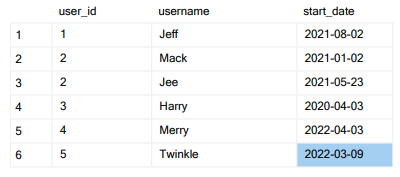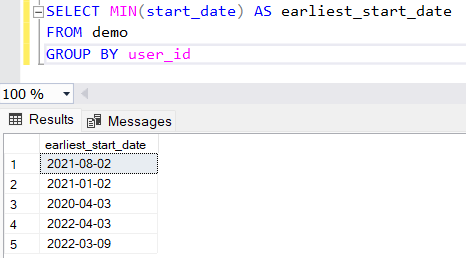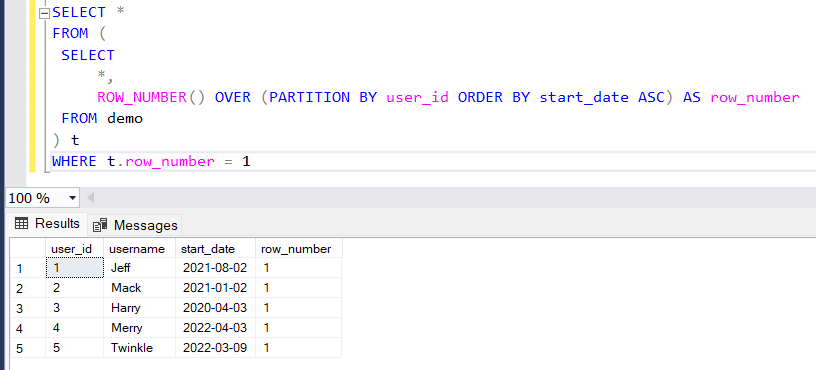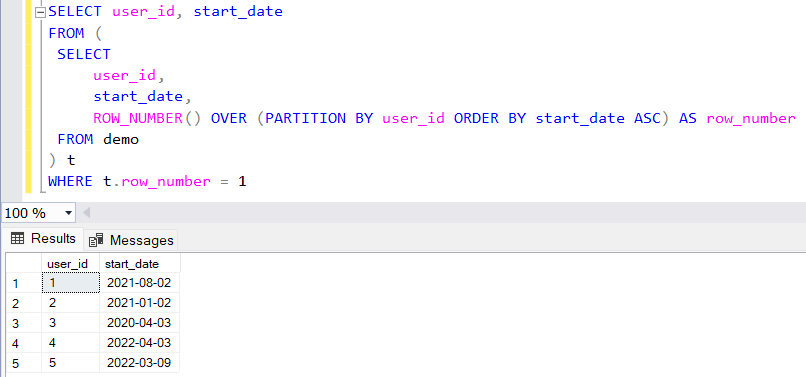GeeksforGeeks App
Open AppBrowser
Continue

# How to Select the First Row of Each GROUP BY in SQL?

The GROUP BY Statement in SQL is used to arrange identical data into groups with the help of some functions. To select the first row of each group in SQL, you can use the ‘ GROUP BY ‘ clause with the ‘ MIN ‘ or ‘ MAX ‘ aggregate function.

### Syntax

```SELECT *
FROM yourTable
WHERE columnName = (SELECT MIN(columnName)
FROM yourTable GROUP BY groupColumnName)
```

This query selects all rows from ‘ yourTable ‘ where the value in ‘ columnName ‘ is equal to the minimum value of ‘ columnName ‘ in each group defined by ‘ groupColumnName ‘.

Alternatively, you can use the ‘ ROW_NUMBER() ‘ function to assign a unique number to each row within each group, and then select the rows with a ‘ ROW_NUMBER ‘ of 1:

Query:

```SELECT *
FROM (
SELECT *, ROW_NUMBER() OVER (PARTITION BY
groupColumnName ORDER BY columnName) AS row_number
FROM yourTable
) AS t
WHERE t.row_number = 1```

## Steps:

Steps 1: To select the first row of each group in SQL, you can use the ‘ GROUP BY ‘ clause in combination with an aggregate function such as ‘ MIN() ‘ or ‘ MAX() ‘. For example, the following query selects the earliest start date for each group of records with the same ‘ user_id ‘:Database

Query:

```SELECT MIN(start_date)
AS earliest_start_date
FROM your_table
GROUP BY user_id```

This query uses the ‘ MIN() ‘ function to find the earliest start date for each group of records with the same ‘ user_id ‘. The ‘ GROUP BY ‘ clause groups the records by ‘ user_id ‘, and the ‘ SELECT ‘ statement returns the minimum start date for each group.

Output:Step 2: You can also use the ‘ ROW_NUMBER() ‘ function to select the first row of each group. For example:

Query:

```SELECT *
FROM (
SELECT
*,
ROW_NUMBER() OVER (PARTITION BY user_id
ORDER BY start_date ASC) AS row_number
FROM your_table
) t
WHERE t.row_number = 1```

This query uses the ‘ ROW_NUMBER() ‘ function to assign a unique number to each row in each group of records with the same ‘ user_id ‘. The ‘ PARTITION BY ‘ clause specifies the column or columns by which the records should be grouped, and the ‘ ORDER BY ‘ clause specifies the ordering of the rows within each group. In this case, the records are ordered by ‘ start_date ‘ in ascending order.

Finally, the outer ‘ SELECT ‘ statement filters the result set to include only rows where the ‘ row_number ‘ is 1, which corresponds to the first row in each group.

Output:Step 3: You can modify this query to return only specific columns by replacing the ‘ * ‘ in the ‘ SELECT ‘ clause with a comma-separated list of the columns you want to include in the result set. For example, the following query returns the ‘ user_id ‘ and ‘ start_date ‘ columns for the first row of each group:

Query:

```SELECT user_id, start_date
FROM (
SELECT
user_id,
start_date,
ROW_NUMBER() OVER (PARTITION BY user_id
ORDER BY start_date ASC) AS row_number
FROM your_table
) t
WHERE t.row_number = 1```

Output:My Personal Notes arrow_drop_up